# Accuracy Specifications - Reading it Right with Range

The accuracy of a measurement instrument varies with the range over which a reading is measured. Not all instrument manufacturers specify accuracy and ranges in the same manner. This article explores the impact of range definitions on measurement accuracy and how one can be mindful when comparing accuracy across instruments.

Basic accuracy represents the best possible accuracy of a measuring device. In the past, this was based on the DC specification, but today it is specified and optimized for the AC power frequency. Manufacturers of power measurement devices often feature this term on their data sheets. Since “basic accuracy” does not have a standard definition, it is open to skewed and often misleading interpretations such as the following examples:

• Some manufacturers specify basic accuracy based on typical or best case data instead of a guaranteed performance.
• Others are not limited to voltage and current specifications since power measurement range results from the multiplication of the voltage and current ranges. But this ignores the effects of power factor, phase angle error; crest factor, temperature range, warm up time, stability period, common mode rejection ratio, etc.
• Some manufacturers only account for uncertainty in a reading and do not take into account the influence of the measurement range error.

#### Accuracy and measurement range

Since the accuracy of a power measurement varies with the measurement range, any specified accuracy value should be accompanied by the range over which it is valid. Without this, a user cannot determine whether the accuracy values are valid only at a single point, a few points of a measurement range or the entire range.

But what if this range is specified in different ways in different instruments? For example, the accuracy of an instrument, when its range is specified in peak values, appears to look far more impressive than when using root mean square (rms) values. How can we make an ‘apples to apples comparison’ of voltage and current uncertainties across different instruments? And what of the adverse effect this can have when calculating active power? The multiplication of voltage, current, and the power factor with higher crest factors will dramatically magnify this effect.

#### How the measurement range affects accuracy specifications

Power meter manufacturers are largely in agreement with the definition of accuracy in the form “x% of the measured value + y% of the measuring range” where the power measurement range is the multiplication of the voltage and current measuring ranges. In order to make realistic comparisons, one should realise that the component “y% of the measuring range” also has different definitions. While some manufacturers use nominal rms range as reference for defining their uncertainty specifications, others use the maximum measurable peak value.

Understanding these definitions is key to making consistent comparisons between different instruments.

#### Understanding measurement range

In the days of purely analog measurement technology, the definition of the range was clear. If the range of an rms voltage meter was set at 250 V, the full scale value was 250 V. For all accuracy data, including accuracy class and basic accuracy, the reference maximum was 250 V.

For digital measuring instruments however, more definitions need to be understood:

1. Selection range, rated range or nominal range corresponds to 100% of the rms value of the range. It is used for accuracy references to the range and selected by an engineer on the instrument based on the needs of his or her applications.
2. Specification range is the range within which the accuracy specifications are valid. However, not every manufacturer can guarantee these accuracy specifications because this requires an ISO17025 accredited calibration.
3. Full scale value is the maximum display value above which the measured values cannot be displayed by an instrument.
4. Blanking value is the minimum display value below which an instrument is unable to display readings.
5. Maximum measurable peak value is the value above which amplitudes are cut off due to dynamic limit of the Analog to Digital (A/D) converter. This value determines how well distorted signals can be measured correctly without clipping and usually corresponds to the set crest factor times the nominal/rated rms range.
6. Maximum permitted input is the maximum peak and rms values of voltage and current that an instrument can withstand before it is damaged.

The figure below depicts the key parameters for a signal at 300 Vrms nominal range for a Yokogawa WT1800E. At 45-66 Hz, the voltage uncertainty specification of 0.03% reading and 0.05% range is valid and guaranteed from 1% to 110% (yellow region up to 330 Vrms) of the set nominal range. The maximum measurable peak value is 3 times the nominal range, resulting in the widest dynamic range to capture distorted waveforms.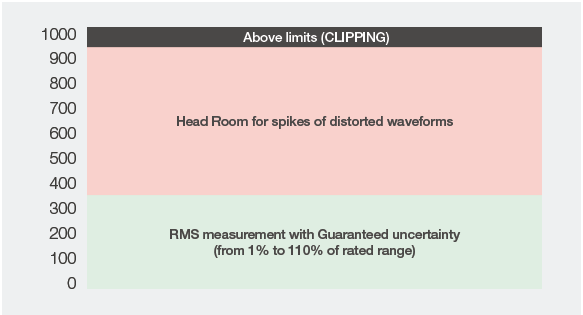Figure 1. Measurement ranges with rated range reference.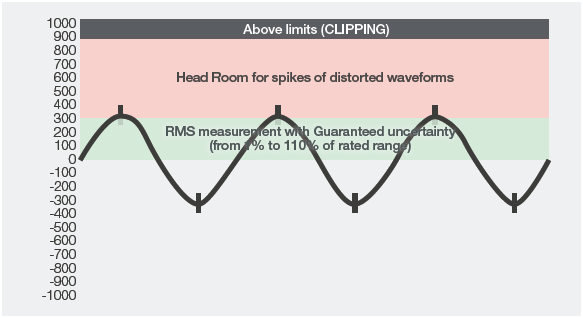Figure 2. Waveform measurement with rated range reference.

#### Choosing a reference for specifying accuracy – Peak or rms?

Accuracy specifications are defined using a reference value of the measurement range and as discussed earlier, manufacturers may choose between the nominal range and the maximum measureable peak for this purpose. In the example below, a Yokogawa WT5000 uses the nominal (rms derived) range as the reference to specify the range over which its accuracy specifications are valid. The values are calculated at 45-66 Hz and a power factor of 1. The same specifications when derived using the peak values as the reference deceptively look far more impressive as highlighted in Table 1.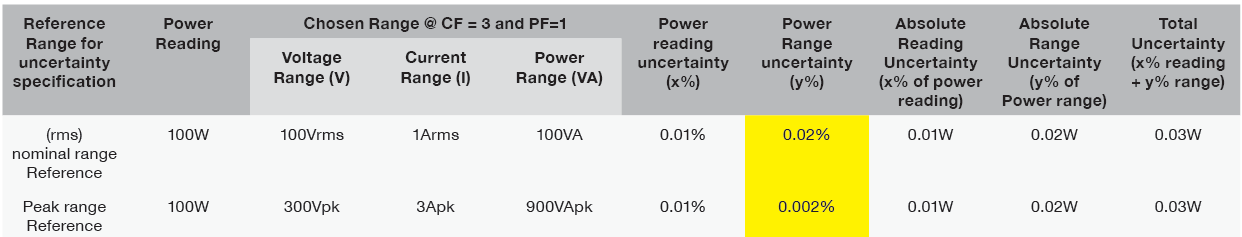Table 1. Range uncertainties of the WT5000 specified using nominal range reference and peak range as reference.

The explanation for this is very simple: When converting the power uncertainty from the rms nominal range reference (0.02%), to that derived from a peak value reference, the respective range crest factors for both the voltage and current ranges (3 in this example) need to be taken into account. This results in a division of the relative power range uncertainty by a factor of 9 giving 0.0022% (without affecting accuracy).

Thus a power measurement when using a voltage range of 100 Vrms and a current range of 1 Arms would appear to have a lower accuracy using the basic specifications than when using a voltage range of 300 Vpk and a current range of 3 Apk even though the absolute uncertainty remains the same (Figure 3).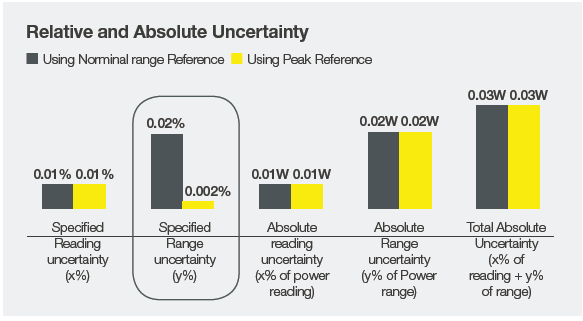Figure 3. Relative and absolute uncertainties when using nominal range reference and peak value reference.

To compare instruments using these two different references, one could convert the uncertainty of a nominal range reference instrument into its peak reference equivalent by a factor as shown above. Alternatively one could calculate and compare the absolute uncertainties while considering the impacts of specifying them in nominal rms range or maximum peak values.

#### Why do some manufacturers use peak value as reference?

Using peak values as reference for uncertainty specifications make the specifications appear deceptively impressive as demonstrated in the previous section. Thus an uncertainty specification of 0.005% is not necessarily more impressive than a 0.05% specification. They could just be using different reference values. A better yardstick to compare the accuracy of instruments would be to calculate the absolute uncertainties of reading and range.

Peak value definitions also distract from the absolute uncertainty values of an instrument or a poor dynamic range for capturing signal distortions. Instruments with low, inconsistent or unspecified crest factors make it difficult to ensure sufficient headroom (figure 5) to capture distortions and spikes in an input signal and may even clip the peaks of signals during measurements.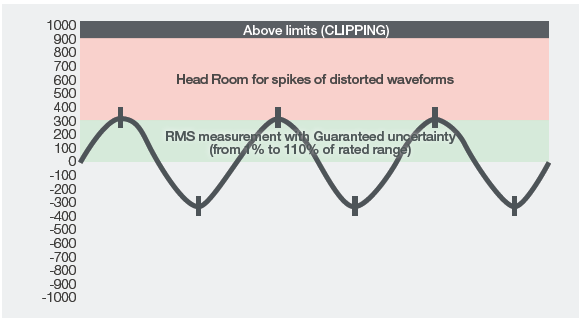Figure 4. Instrument with sufficient headroom to capture distortions.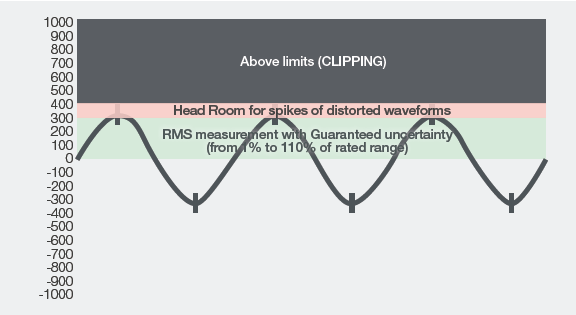Figure 5. Instrument with insufficient headroom to capture distortions.

#### Is it better to use nominal/rated range as reference?

Among the advantages of using nominal or rated rms range as the reference is that it is a broadband measuring method that does not differentiate between different frequencies. This makes it easy to determine measurement uncertainty at specific frequencies for different amplitudes. As we have learned in previous sections, the accuracy of an instrument is different at different ranges. The closer a reading is to its full scale measurement range, the more accurate it is.

The best basic accuracy of an instrument is achieved when the reading is at 100% of the range. Uncertainty = x% reading + y% range = x% reading + y% reading (since range=reading).

But when reading is at 50% of the range, i.e. range = 2 x reading, the uncertainty increases: Uncertainty = x% reading + y% range = x% reading + y% (2x reading).

Table 2 shows the effect of choosing different ranges on the overall accuracy of a reading.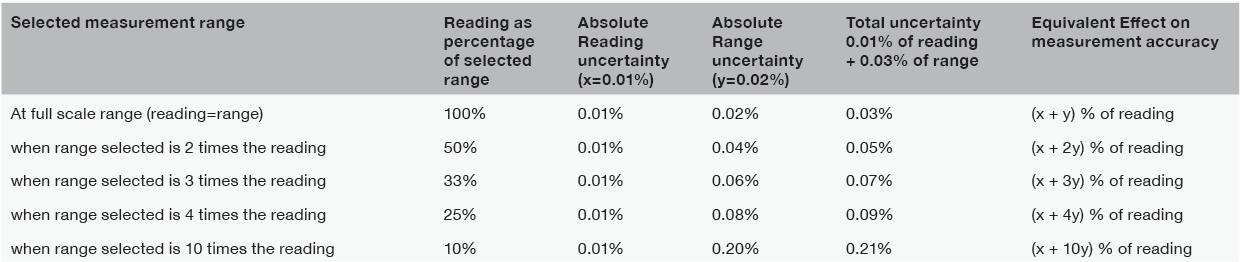Table 2. Measurement uncertainty of Yokogawa WT5000 at various amplitudes, with nominal range value as reference. (Valid at 45-66Hz; 23±5° and Power factor 1).

There is thus a simple relationship between the set range and measurement accuracy when using nominal range as reference. The advantage is even more evident when looking at the accuracy specifications of a harmonic analysis where results are, as a matter of principle, amplitudes of single sinusoidal oscillations with a crest factor of 1.414. Here, both rms value and peak value are always lower than the maximum peak of the measurement range.

#### Conclusion - Transparency for trust

We have now seen that without a specified validity range, an engineer cannot be sure at which points an instrument is accurate. A reliable measurement instrument offers a transparent way to assess its accuracy specifications so that users can assess its suitability to the unique accuracy needs of their applications.

Since there is no standard to define accuracy specifications, a fair comparison can be difficult. The only solution then is to compare the accuracy of instruments on calculated absolute uncertainties while considering the impacts of specifying them in nominal rms range or maximum peak values. What is indeed more practical is the use of guaranteed measurement uncertainties which take into account the effects of reading and range components.

### Related Products & Solutions

#### PX8000 - Precision Power Scope

• Transient/Start-up Waveform Capture & Power Analysis
• Harmonics, Cycle by cycle and FFT analysis

#### Power Analyzers and Power Meters

Measure characteristics of devices that generate, transform or consume electricity. Also called power meters or wattmeters, these devices measure parameters such as true power (watts), power factor, harmonics, and efficiency.

Top